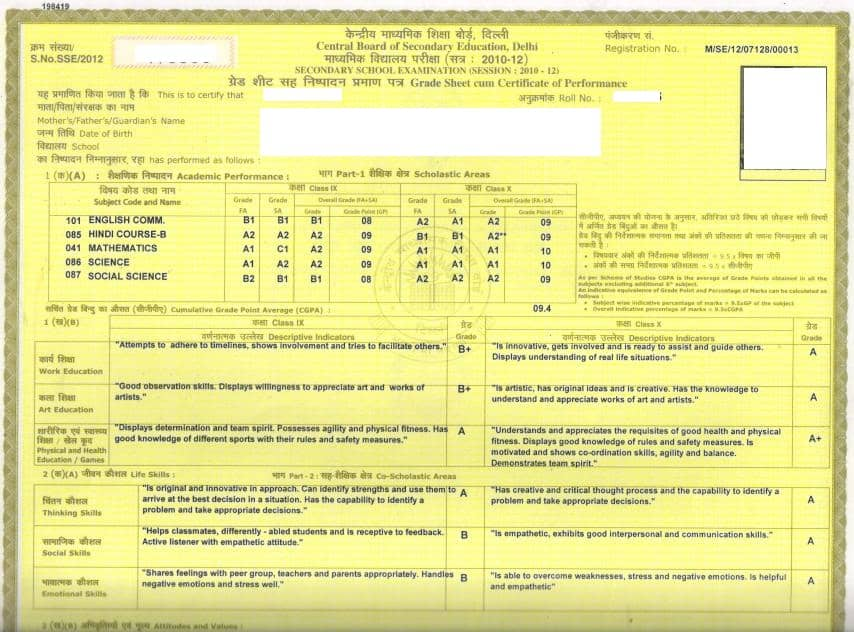1. /
2. CBSE
3. /
4. Class 10
5. /
6. What is CGPA

# What is CGPA

What is CGPA in CBSE system of evaluation ?

How CGPA is calculated ?

How to calculate percentage % with CGPA?

Cumulative Grade Point Average(CGPA) is the average of Grade Points obtained in all the subjects excluding 6th additional subject as per Scheme of Studies.

Cumulative Grade Point Average(CGPA) would also be reflected in the  Grade card so there is no need to calculate it. However, you can use the following formula to calculate overall CGPA :

CGPA = sum of Grade points (main 5 subjects) divided by 5

for example: 9+9+10+10+9/5 =47/5=9.4 (CGPA)

To calculate percentage from CGPA, simply multiply it with 9.5 such as

CGPA=9.4, overall percentage is : 9.4 x 9.5 = 89.3 % Marks.

NOTE : In class 10 Report card, Grades of class 9 subjects are also mentioned but CGPA and percent is calculated from class 10 marks only.  Sample Grade card is given below :### Test Generator

Create question paper PDF and online tests with your own name & logo in minutes.### myCBSEguide

Question Bank, Mock Tests, Exam Papers, NCERT Solutions, Sample Papers, Notes

### 8 thoughts on “What is CGPA”

1. when wiLL the 10th cBSE result wilL anNounce..??

2. Results date kya hai CBSE 10th ka _/_ ?

3. 10 class result declared om 30. May

4. Hi would like to know if information technology is included in calculating the cgpa for grade 10 for the year 2014-2015

5. u mean class 9th marks don’t matter in calculation of CGPA.It won’t affect our 10th class marks?